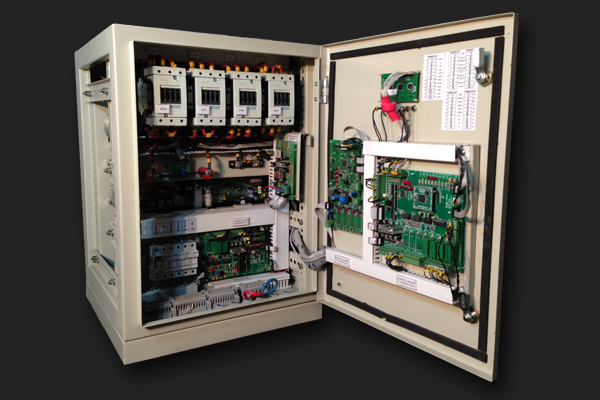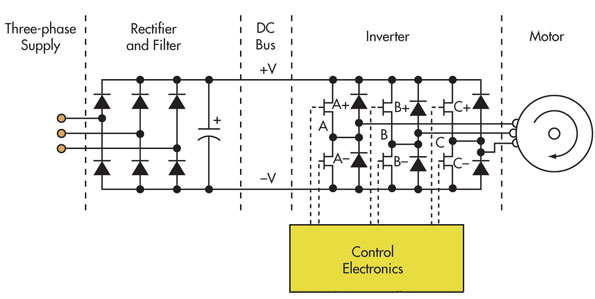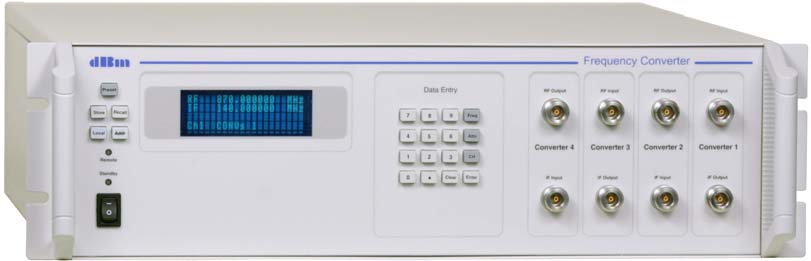# A Guide to Efficient Energy Management By Frequency Converters

Have you ever wondered how your industrial processes could be made more efficient and cost-effective? Enter the world of frequency converters. These electronic devices have revolutionized the way we control the speed of electric motors and adapt the power supply to different operating conditions. By converting the frequency of an electrical signal from one value to another, frequency converters enable us to save energy, reduce mechanical stress on equipment, and improve process control. In this guide, we'll explore the ins and outs of frequency converters, their different types, applications, advantages, and maintenance, as well as provide tips on how to select the right converter for your specific needs. So, power up your processes, and let's get started!Fig 1. Frequency Converter

## What is a Frequency Converter?

In general, a converter is a device or software that converts something from one form or state to another. The specific meaning of "converter" can vary depending on the context in which it is used. A few examples are electrical converters, file converters, unit converters, and currency converters. In general, converters are used to help people or systems communicate or interact more effectively by converting information from one form to another.

A frequency converter is a type of electrical converter that converts the frequency of an alternating current (AC) power source from one frequency to another. This is typically done in situations where the frequency of the power source needs to be changed to match the requirements of a particular device or system.

Frequency converters are often used in industrial applications, such as in manufacturing processes or the operation of machinery. They can also be used in other applications, such as in power grids, where they can help to stabilize the frequency of the electricity supply.

There are two main types of frequency converters:

• AC-to-AC converters
• DC-to-AC converters

AC-to-AC converters are used to convert the frequency of AC power sources, while DC-to-AC converters are used to convert the frequency of DC power sources to AC power.

Frequency converters can be either static or rotary. Static frequency converters use solid-state electronic components to convert the frequency of the power source, while rotary frequency converters use electromechanical components such as motors and generators to achieve the same result.

Overall, frequency converters are an important technology that allows devices and systems to operate more efficiently and effectively by providing power at the required frequency.

## How Many Types does a Frequency Converter Have?

There are several types of frequency converters, which can be classified based on their output frequency and application. Here are some common types of frequency converters:

1. AC-to-AC frequency converters: These converters are used to change the frequency of an AC power source to a different frequency. They can be used for a wide range of applications, including motor control, power conversion, and energy savings.
2. DC-to-AC frequency converters: These converters are used to convert DC power to AC power at a specific frequency. They are commonly used in renewable energy systems, such as solar and wind power, to convert DC power to AC power for use in the grid.
3. Static frequency converters: These converters are designed to convert the frequency of AC power without the use of moving parts. They are commonly used in aerospace and defense applications, as well as in industrial automation.Fig

Fig 2. The static frequency converter

### What is the DC Bus in a Static Frequency Converter?

In a static frequency converter (SFC), the DC bus refers to the direct current (DC) electrical system that is used as an intermediate energy storage and distribution system within the converter. The DC bus typically consists of one or more capacitors or batteries that store the converted DC power. These components provide the necessary energy for the inverter to generate the output AC power at the desired frequency. The size and capacity of the DC bus depend on the power requirements of the SFC and the duration of operation required.

 Measurement Reason W Total power in dc bus. Can be used in efficiency calculations. Arms RMS charging current in d.c. bus. Useful for sizing conductors or fuses. AHO DC component of current in dc bus. This is smaller than Arms. VHO Mean voltage across storage capacitor. Vpk Peak voltage across storage capacitor.

Table 1. Critical Measurement parameters for frequency converter DC bus.

1. Rotary frequency converters: These converters use a motor generator set to convert the frequency of AC power. They are commonly used in marine and offshore applications, as well as in aviation and military applications.
2. Variable frequency drives (VFDs): These converters are used to control the speed of AC motors by varying the frequency of the power supplied to the motor. They are commonly used in industrial automation applications, such as conveyor systems, pumps, and fans.

### Frequency Converter Formulas

Here are some common formulas used in frequency converters:

#### Frequency Conversion Formula

f2 = f1 x (N2/N1)

• Where f1 is the input frequency, f2 is the output frequency, N1 is the number of poles on the input motor, and N2 is the number of poles on the output motor.

For example, if a frequency converter is used to control the speed of a three-phase AC motor with six poles at an input frequency of 50 Hz and an output frequency of 30 Hz, the number of poles on the output motor can be calculated as

N2 = (f2/f1) x N1 = (30/50) x 6 = 3.6

Since the number of poles on a motor must be an integer value, the output motor would be selected to have four poles instead of 3.6.

#### Motor Speed Formula

N = (120 x f) / P

• Where N is the motor speed in revolutions per minute (RPM), f is the frequency of the power supplied to the motor in hertz (Hz), and P is the number of poles on the motor.

For example, if a three-phase AC motor with six poles is supplied with power at a frequency of 60 Hz, the motor speed can be calculated as

N = (120 x 60) / 6 = 1200 RPM

#### Voltage Conversion Formula

V2 = V1 x (f2/f1)

• Where V1 is the input voltage, V2 is the output voltage, f1 is the input frequency, and f2 is the output frequency.

For example, if a frequency converter is used to convert an input voltage of 220 volts at an input frequency of 50 Hz to an output frequency of 60 Hz, the output voltage can be calculated as

V2 = V1 x (f2/f1) = 220 x (60/50) = 264 volts

These are just a few examples of the formulas used in frequency converters. The specific formulas used will depend on the application and the design of the frequency converter.

 Frequency conversion formula f2 = f1 x (N2/N1) Motor speed formula N = (120 x f) / P Voltage conversion formula V2 = V1 x (f2/f1)

Table 2. Frequency converter formulas at a glance

## What is an RF Frequency Converter?

An RF (Radio Frequency) frequency converter is a type of electronic device that is used to convert a signal from one RF frequency to another. This type of converter is commonly used in radio communication systems and other RF applications.

RF frequency converters typically consist of two main parts:

• A local oscillator
• A mixer

The local oscillator generates a signal at a specific frequency, which is then mixed with the input signal to produce an output signal at a different frequency. The difference between the two frequencies is known as the intermediate frequency (IF).

The IF signal can then be further processed or transmitted as needed. RF frequency converters can be designed to work with a variety of input and output frequencies, depending on the specific requirements of the application.

RF frequency converters are used in a variety of applications, such as in wireless communication systems, radar systems, and electronic warfare systems. They can also be used in satellite communication systems, where they are used to convert the frequency of signals sent from the satellite to a frequency that can be received by ground-based equipment.Fig 3. RF frequency converter

## What is Voltage to Frequency Converter?

A voltage-to-frequency converter is an electronic device that converts an input voltage signal into a corresponding output frequency signal. The frequency of the output signal is proportional to the input voltage level, and this relationship can be used to transmit or measure analog signals using digital circuits.